# 【机器学习】--Python机器学习库之Numpy

2018/04/06 20:39

NumPyNumerical Python的缩写）是一个开源的Python科学计算库。使用NumPy，就可以很自然地使用数组和矩阵 NumPy包含很多实用的数学函数，涵盖线性代数运算、傅里叶变换和随机数生成等功能

1、背景--为什么使用Numpy？

a) 便捷：

b) 性能：

NumPy中数组的存储效率和输入输出性能均远远优于Python中等价的基本数据结构（如嵌套的list容器）。其能够提升的性能是与数组中元素的数目成比例的。对于大型数组的运算，使用NumPy的确很有优势。对于TB级的大文件，NumPy使用内存映射文件来处理，以达到最优的数据读写性能。

c) 高效：

NumPy的大部分代码都是用C语言写成的，这使得NumPy比纯Python代码高效得多。

当然，NumPy也有其不足之处，由于NumPy使用内存映射文件以达到最优的数据读写性能，而内存的大小限制了其对TB级大文件的处理；此外，NumPy数组的通用性不及Python提供的list容器。因此，在科学计算之外的领域，NumPy的优势也就不那么明显。

2、Numpy的安装

（1） 官网安装。http://www.numpy.org/

（2） pip 安装pip install numpy

（3） LFD安装，针对windows用户http://www.lfd.uci.edu/~gohlke/pythonlibs/

3、numpy 基础：

NumPy的主要对象是同种元素的多维数组。这是一个所有的元素都是一种类型。

NumPy中维度(dimensions)叫做轴(axes)，轴的个数叫做秩(rank)

NumPy的数组类被称作 ndarray 。通常被称作数组。

ndarray.ndim(数组轴的个数,轴的个数被称作秩)

ndarray.shape(数组的维度。这是一个指示数组在每个维度上大小的整数元组。例如一个nm列的矩阵，它的shape属性将是(2,3),这个元组的长度显然是秩，即维度或者ndim属性)

ndarray.size数组元素的总个数，等于shape属性中元组元素的乘积

ndarray.dtype一个用来描述数组中元素类型的对象，可以通过创造或指定dtype使用标准Python类型。另外NumPy提供它自己的数据类型）。

4、Numpy的数据类型：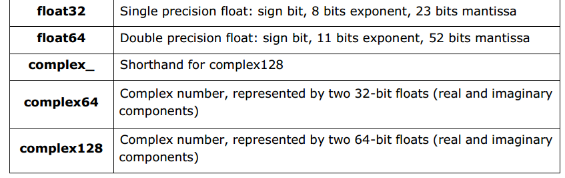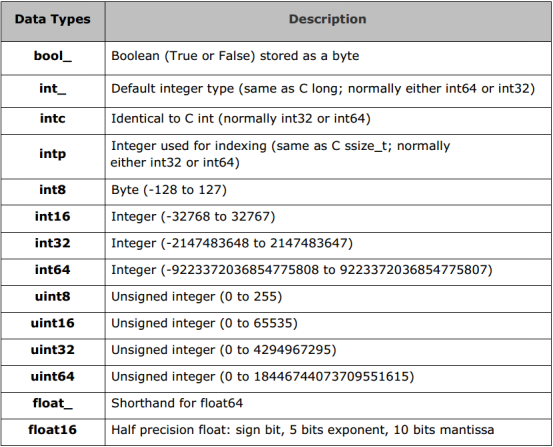import numpy as np
a = np.dtype(np.int_)     #  np.int64, np.float32 …
print(a)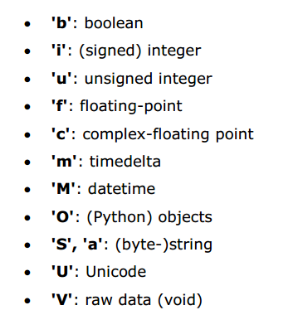int8, int16, int32,int64 可以字符串’i1’, ‘i2’,’i4’, ‘i8’代替，其余的以此类推。

import numpy as np
a = np.dtype('i8')   # ’f8’, ‘i4’’c16’,’a30’(30个字符的字符串), ‘>i4’…
print (a)

import numpy as np
a = np.array([[1,2,3], [4, 5, 6]], dtype=int)
print(a.shape)       #  a.ndim, a.size, a.dtype
import numpy as np
a = np.array([(1,2,3), (4, 5, 6)], dtype=float)
print(a.shape)      #  a.ndim, a.size, a.dtype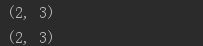import numpy as np
a = np.arange(10).reshape(2, 5) # 创建2行5列的二维数组，
# 也可以创建三维数组，
b = np.arange(12).reshape(2,3,2)
print(a)
print(b)

a = np.array([[[1,2,3], [4, 5, 6], [7, 8, 9]]])
b = np.array([[[1,2,3]], [[4, 5, 6]], [[7, 8, 9]]])
print(a.shape)
print(b.shape)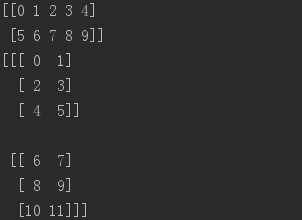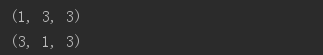代码三：基本运算

import numpy as np
a = np.random.random(6)
b = np.random.rand(6)
c = np.random.randn(6)
print(a-b)                    # print(a+b),print(a*c) …
print(np.dot(a,b))          #复习矩阵乘法

# Numpy 随机数模块np.random.random, np.random.randn, np.random.rand的比较

1）rand 生成均匀分布的伪随机数。分布在（0~1）之间

2）randn 生成标准正态分布的伪随机数（均值为0，方差为1）。

import numpy as np
a = np.ones((2,3))
b = np.zeros((2,3))
a*=3
b+=a
print(a)
print(b)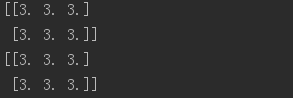代码四：常用函数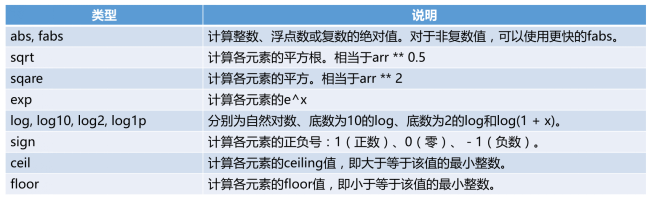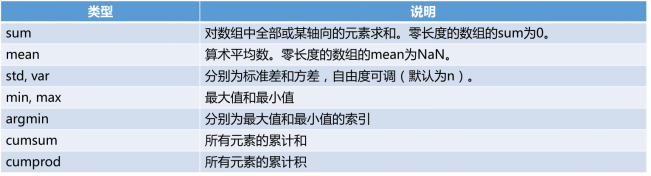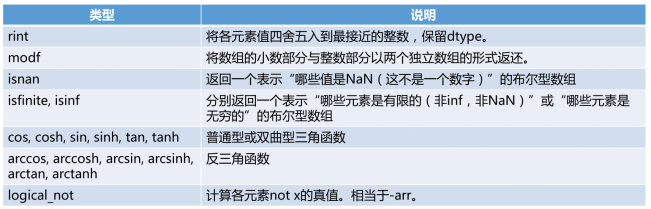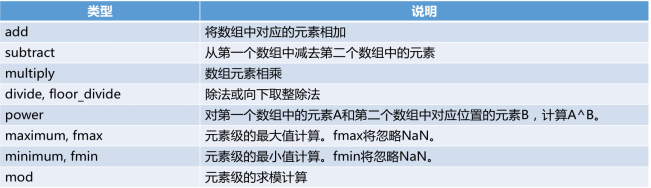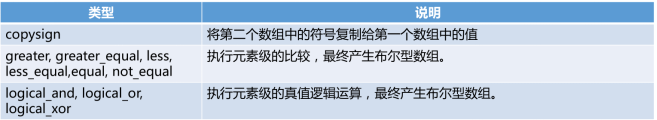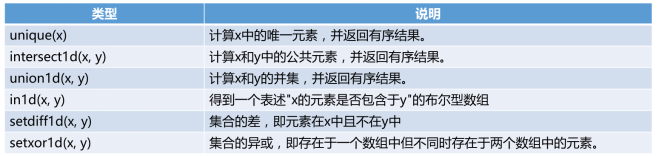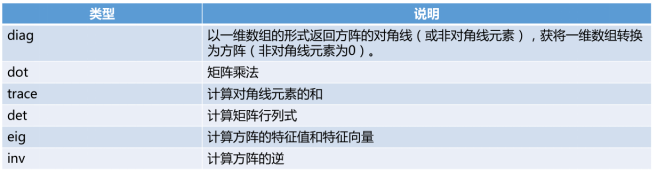import numpy as np
a = np.arange(10)**3
print(a)
print(a[2:5])
a[:6:2] = -1000
print(a)
print(a[ : :-1])

[x:y:z]切片索引,x是左端,y是右端,z是步长,在[x,y)区间从左到右每隔z取值,默认z为1可以省略z参数.

b = np.arange(20).reshape(5,4)
print(b)
print(b[2,3])
print(b[0:5, 1])
print(b[ : ,1])
print(b[1:3, : ])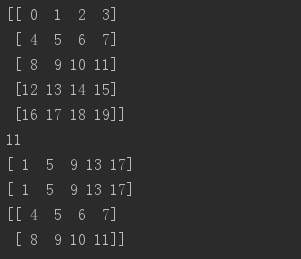#当少于轴数的索引被提供时，丢失的索引被认为是整个切片

b[-1]    #相当于b[-1,:] 最后一行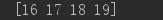# b[i] 中括号中的表达式被当作 i 和一系列 : ，来代表剩下的轴。NumPy也允许你使用“点”像 b[i,...] 。

#点 (…)代表许多产生一个完整的索引元组必要的分号。如果x是

#秩为5的数组(即它有5个轴)，那么:

x[1,2,…] 等同于 x[1,2,:,:,:],

x[…,3] 等同于 x[:,:,:,:,3]，

x[4,…,5,:] 等同 x[4,:,:,5,:].

c = np.arange(12).reshape(2,3,2)
print(c)
c
c[2,1]    # 等价于c
c[2,1,1]  # 等价于c

f = np.arange(12).reshape(3, 4)
print(f)
g = f>4
print(g)
print(f [g])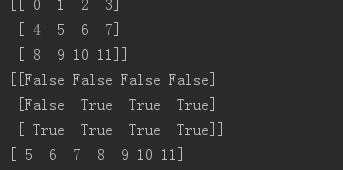h = np.arange(12).reshape(3,4)
print(h)
for i in h:
print(i)
for i in h.flat:
print(i)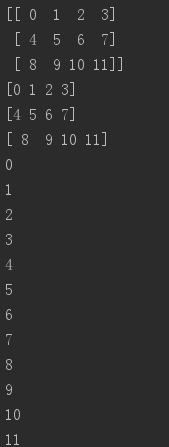np.flatten()返回一个折叠成一维的数组。但是该函数只能适用于numpy对象，即array或者mat，普通的list列表是不行的。

a = np.array([[1,2], [3, 4], [5, 6]])

print(a.flatten())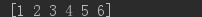代码六：形状操作

ravel(), vstack(),hstack(),column_stack,row_stack, stack, split, hsplit, vsplit

import numpy as np
#增加维度
a = np.arange(5)
print(a[:, np.newaxis])
print(a[np.newaxis, :])
print(np.tile([1,2], 2))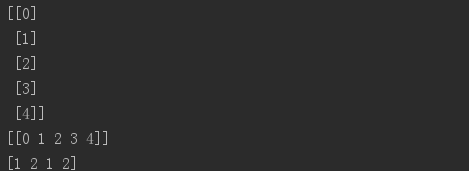a = np.arange(10).reshape(2,5)
print(a)
print(a.ravel())
print(a.resize(5,2))
b = np.arange(6).reshape(2,3)
c = np.ones((2,3))
d = np.hstack((b,c))
print(b)
print(c)
print(d)# hstack：horizontal stack 左右合并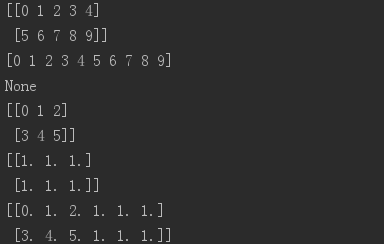e = np.vstack((b,c))
print(e)# vstack: vertical stack 上下合并
f = np.column_stack((b,c))
g = np.row_stack((b,c))
h = np.stack((b, c), axis=1)      # 按行合并
i = np.stack((b,c), axis=0)       # 按列合并
j = np.concatenate ((b, c, c, b), axis=0)   #多个合并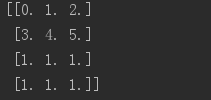0
0 收藏

### 作者的其它热门文章0 评论
0 收藏
0